Win up to 100% scholarship on Aakash BYJU'S JEE/NEET courses with ABNAT Win up to 100% scholarship on Aakash BYJU'S JEE/NEET courses with ABNAT

# Modulus and Conjugate of a Complex Number

The modulus of a complex number gives the distance of the complex number from the origin in the Argand plane, whereas the conjugate of a complex number gives the reflection of the complex number about the real axis in the Argand plane. In this section, we will discuss the modulus and conjugate of a complex number, along with a few solved examples.

## Conjugate of a Complex Number

The conjugate of a complex number z = x + iy is denoted by

$$\begin{array}{l}\bar z = x – iy\end{array}$$
. It is the reflection of the complex number about the real axis on Argand’s plane or the image of the complex number about the real axis on Argand’s plane. If we replace the ‘i’ with ‘- i’, we get a conjugate of the complex number.

The geometrical representation of the complex number is shown in the figure given below: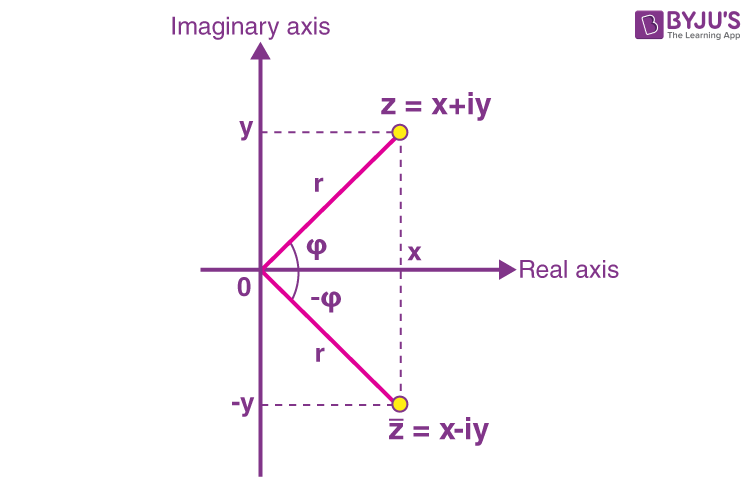### Properties of the Conjugate of a Complex Number

Below are some properties of the conjugate of complex numbers, along with their proof.

(1)

$$\begin{array}{l}\overline{z_1 \pm z_2} = \bar z_1 \pm \bar z_2\end{array}$$

Proof: Let z1 = a + ib and z2 = c + id

Then,

$$\begin{array}{l}\overline{z_1 \pm z_2} = \overline{{a+ib} \pm {c+id}}\end{array}$$
$$\begin{array}{l} =\overline{{a \pm c + ib \pm id}}\end{array}$$

= a ± c – i(b ± d)

= a – ib ± c ± id

= a – ib ± (c – id)

$$\begin{array}{l} =\bar z_1 \pm \bar z_2\end{array}$$

(2)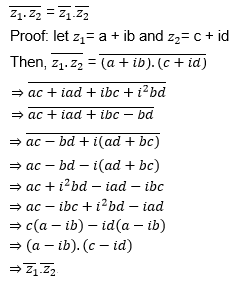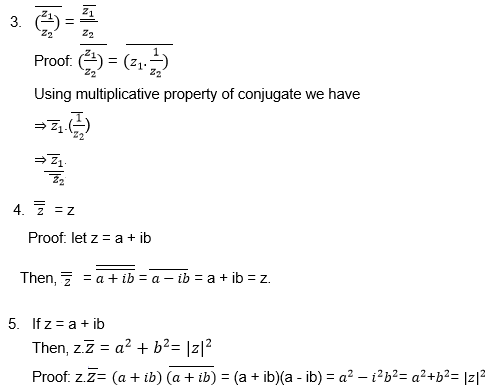Points to Remember: $$\begin{array}{l}z + \bar z = 2\ Re(z)\end{array}$$ $$\begin{array}{l}z – \bar z= 2i\ Im(z)\end{array}$$ If z lies in the 1st quadrant, then $$\begin{array}{l}\bar z\ \text{will lie in the 4th quadrant, and}\ – \bar z\end{array}$$ will lie in the 2nd quadrant. If x + iy = f(a + ib) then x – iy = f(a – ib) Further, g(x + iy) = f(a + ib) ⇒ g(x – iy) = f(a – ib).

## Modulus of a Complex Number

The modulus of the complex number is the distance of the point on the Argand plane representing the complex number z from the origin.

Let P is the point that denotes the complex number, z = x + iy.

Then, OP = |z| = √(x2 + y2 ).

 Note: 1. |z| > 0. 2. All the complex numbers with the same modulus lie on the circle with the centre origin and radius r = |z|.

### Properties of Modulus of Complex Number

Below are a few important properties of the modulus of a complex number and their proofs.

(i) |z1 z2| = |z1||z2|

Proof: let z1= a + ib and z2 = c + id

Then, |z1 z2| = |(a + ib)(c + id)|

⇒ |ac + iad + ibc + i2bd|

⇒ |ac + iad + ibc – bd|

⇒ |ac – bd + i(ad + bc)|

⇒ (ac)2+ (bd)2 – 2abcd + (ad)2 + (bc)2 + 2abcd

⇒ a2 c2 + b2 d2 + a2 d2+ b2 c2

⇒ a2 c2 + b2 c2 + b2 d2 + a2 d2

⇒ (a2 + b2)c2 + (b2 + a2)d2

⇒ (a2 + b2) (c2 + d2)

⇒ |z1||z2|.

(ii) |z/ z2 | = (|z1|) / (|z2|).

Proof: |z1/z2 | = |z1 . 1/z2 |

Using the multiplicative property of modulus, we have

⇒ |z1| |1/z2|

⇒ |z1| 1/(|z2|)

⇒ (|z1 |) / (|z2|).

 Some other important results: Triangle inequalities: |z1 + z2| ≤ |z1| + |z2| |z1 + z2| ≥ |z1| – |z2| |z1 – z2| ≥ |z1| – |z2|.

## Examples on Modulus and Conjugate of a Complex Number

Example 1: Find the conjugate of the complex number z = (1 + 2i)/(1 – 2i).

Solution: z = (1 + 2i)/(1 – 2i)

Rationalising given the complex number, we have

⇒ z = ((1 + 2i)/(1 – 2i) )× (1 + 2i)/(1 + 2i)

⇒ z = (1 + 2i)2/(12 – (2i)2)

⇒ z = (1 + 4i2 + 4i)/(1 + 4)

⇒ z = (1 – 4 + 4i)/(1 + 4)

⇒ z = (-3 + 4i)/5

$$\begin{array}{l}\Rightarrow \bar z = \frac{(-3 – 4i)}{5}\end{array}$$

Example 2: Find the modulus of the complex number z = (3 – 2i)/2i

Solution: z = (3 – 2i)/2i

⇒ z = (3 )/2i – 2i/2i

⇒ z = 3/2i – 1

⇒ z = 3i/(2i2 ) – 1

⇒ z = (-3i/2) – 1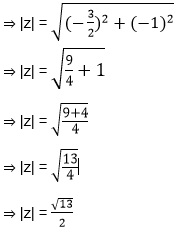Example 3: If z + |z| = 1 + 4i, then find the value of |z|.

Solution: Let z = x + iy

⇒ z + |z| = 1 + 4i

⇒ x + iy + |x + iy| = 1 + 4i

⇒ x + iy + √(x2 + y2 ) = 1 + 4i

⇒ y = 4 and x + √(x2 + y2 ) = 1

⇒ x + √(x2 + 42 ) = 1

⇒ √(x2 + 42 ) = 1 – x

Squaring both sides, we have

⇒ x2 + 42 = 1 + x2 – 2x

⇒ 2x = -15

or x = -15/2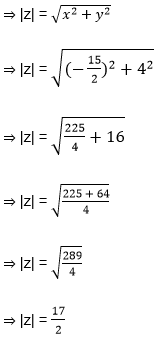Example 4:

$$\begin{array}{l}\text{If }\ |Z-\frac{4}{Z}|=2,\ \text{then the maximum value of |Z| is equal to}\end{array}$$

Solution:

$$\begin{array}{l}|z-\frac{4}{z}|\geq |z|-|\frac{4}{z}|\\ 2\geq |z|-\frac{4}{|z|}\\ 2|z|\geq |z|^{2}-4\\ |z|^{2}-2|z|-4\leq 0\\ |z|\leq \sqrt{5}+1\\\end{array}$$

Example 5: Let (α, β) be real, and z be a complex number. If z2 + αz + β = 0 has distinct roots on the line Re z = 1, then find the necessary condition.

Solution:

Given Re z = 1

Let z1 = x + iy and z2 = x – iy are the roots.

Since Re z = 1, x = 1

Let z2 + αz + β = 0 has roots (1 + iy) and (1 – iy)

So,

z1 z2β

(1 + iy)(1 – iy) = β

1 + y2β

β > 1

$$\begin{array}{l} \beta \in (1,\infty)\\\end{array}$$

Example 6: If ω(≠ 1) is a cube root of unity, and (1 + ω)7 = A + Bω, then find (A, B).

Solution:

$$\begin{array}{l}(1+\omega )^{7}=A+B\omega \\ (-\omega^{2} )^{7}=A+B\omega \\ (-\omega^{2} )=A+B\omega \\ 1+\omega =A+B\omega \\ A=1\\ B=1\\ (A,B)=(1,1)\\\end{array}$$

Example 7: A complex number z is said to be unimodular if |z| = 1. Suppose z1 and z2 are complex numbers such that

$$\begin{array}{l}\frac{|z_1-2z_2|}{|2-z_1\overline{z_{2}}|}\ \text{is unimodular and}\ z_2\ \text{is not unimodular}.\end{array}$$
Then point z1 lies on a _____.

Solution:

$$\begin{array}{l}\frac{|z_1-2z_2|}{|2-z_1\overline{z_{2}}|}=1\\ \Rightarrow |z_1-2z_2|^{2}=|2-z_1{\overline{z_2}}|^{2}\\ \Rightarrow |z_1-2z_2|(\overline{z_1}-2{\overline{z_2}})= (2-z_{1}\overline{z_{2}})(2-\overline{z_{1}}z_{2})\\ |z_1|^{2}+4|z_2|^{2}-4-|z_1|^{2}|z_2|^{2}=0\\ 4[(|z_2|^{2}-1)-|z_1|^{2}(|z_2|^2-1)]=0\\ \Rightarrow |z_1|^{2}-4=0\end{array}$$

⇒ |z1| = 2 is a circle of radius 2 and centre at origin.

Q1

### What do you mean by a complex number?

A complex number is a number in the form of a+ib, where a and b are real numbers and i = √-1.

Q2

### What do you mean by the conjugate of a complex number?

The conjugate of a complex number z = a + ib is given by z̄ = a – ib.

Q3

### Give the equation for the modulus of a complex number.

The modulus of a complex number z is given by |z| = √(x2 + y2).

Q4

### What is z+z̄, if z is purely imaginary?

If z is purely imaginary, then z+z̄ = 0.

Test your knowledge on Modulus And Conjugate Of A Complex Number